# Understanding Functions Flashcards

Understanding Functions Flashcards
1 / 32 0 0
32 cards in set

## Flashcard Content Overview

There are many characteristics of a function, and this flashcard set will cover a lot of them. Using this flashcard set will aid in retention of facts about functions and in just understanding functions in general. It will cover such concepts as domain and range of a function, adding, subtracting, multiplying and dividing functions, composition of functions, graphs of functions, inverse functions, and function transformations. There's also a couple flashcards that allow you to practice applications of these concepts in a real-world context.

Front
Back
Domain of a function

The possible values of x that we can put into the function.

If f (x) = √(x + 2), and g(x) = x - 3, find the domain of f (x) / g(x).

Domain = [-2, 3) ∪ (3, ∞)

Piecewise function

A function that takes more than one expression to define for different intervals of x.

Find f (2)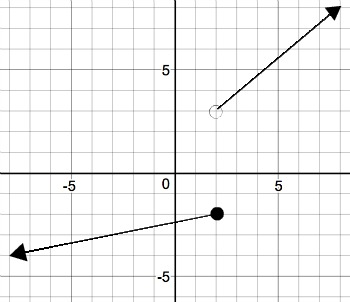-2

Function

An equation or rule that you plug an input into and get an output out of.

Fill in the blank with 'is' or 'is not': The relation {(1, 2), (3, 4), (5, 4), (2, 5)} _____ a function.

is

Relation

A set of ordered pairs relating the first coordinates to the second coordinates by some rule.

If f (x) = 7x - 2, find f (3).

19

The number of products a company sells is f (x) = x2 + 10x + 1000, and the average product price is g(x) = 4x + 50. Find the revenue model.

r(x) = 4x3 + 90x2 + 4500x + 50000

Revenue function

A function that tells how much money a company makes.

f (x) = 3x4 - 2x3 + x - 4

g(x) = 7x2 + 2x + 1

Find f (x) - g(x).

f (x) - g(x) = 3x4 - 2x3 - 7x2 - x - 5

f (x) = 5x2 + 4x

g(x) = 3x

Find f (x) / g(x).

f (x) / g(x) = (5x + 4) / 3, x ≠ 0

f (x) = 2x2 + 5x + 3

g(x) = x2 - 3x - 4

Find f (x) / g(x).

f (x) / g(x) = (2x + 3) / (x - 4), x ≠ -1, 4

Composition of functions

Plugging a function into another function.

f (x) = x2 - 1

g(x) = x + 1

Find f (g(x)).

x2 + 2x

If f (g(x)) = √(x + 4), what is f (x) and g(x)?

f (x) = √(x) and g(x) = x + 4

If f (x) = x3 - 2x and g(x) = |x|, find f (g(-2)).

4

Inverse function

A function that is the opposite of, or undoes, another function.

Rule to determine if f (x) and g(x) are inverse functions

If f (x) and g(x) are inverse functions, then f (g(x)) = g(f (x)) = x.

Horizontal line test

If a horizontal line passes through the graph of a function more than once, then the function does not have an inverse.

How to find an inverse function

1. Replace f (x) with y.

2. Interchange x and y.

3. Solve for y

4. Replace y with f -1 (x).

g(x) in terms of f (x)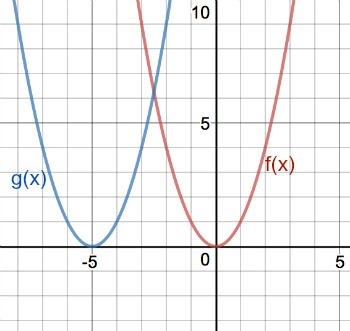g(x) = f (x + 5)

g(x) in terms of f (x)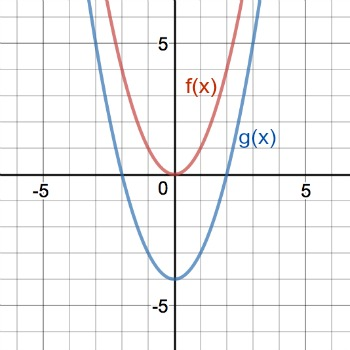g(x) = f (x) - 4

Translation

Sliding a graph along a straight line

Reflection transformation

Reflecting a graph over a line

Reflect the triangle over y = x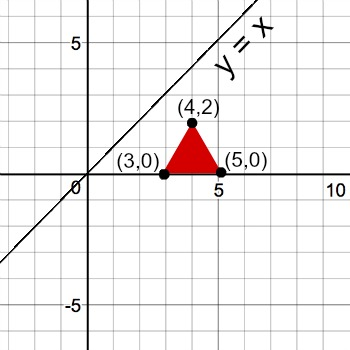Reflected over y = x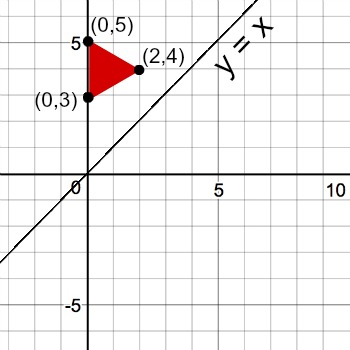f (x) = x2 + 3

g(x) = √(x + 3)

Find the domain of f (g(x))

Domain = all numbers greater than or equal to -3.

(When you find the domain of f(g(x)), you have to take into account the domain of the original function g(x) even though it gets squared in forming the new function.)

Domain of f (x)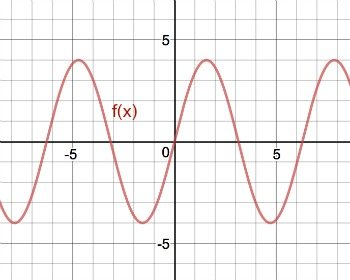All real numbers

Range of a function

Possible output values of the function

Range of f (x)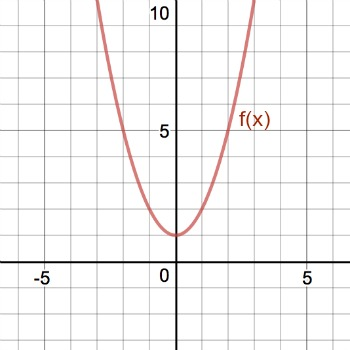Range = all real numbers greater than or equal to 1

Number not in the range of f (x) = 1 / x

0

Domain of f (x) = 4 / (x2 + x - 42)

Domain = all real numbers except -7 and 6

To unlock this flashcard set you must be a Study.com Member.